# Olympiad Test: Problems Based On Figures - 1

## 10 Questions MCQ Test National Cyber Olympiad Class 8 | Olympiad Test: Problems Based On Figures - 1

Description
Attempt Olympiad Test: Problems Based On Figures - 1 | 10 questions in 20 minutes | Mock test for Class 8 preparation | Free important questions MCQ to study National Cyber Olympiad Class 8 for Class 8 Exam | Download free PDF with solutions
QUESTION: 1

Solution:
QUESTION: 2

Solution:
QUESTION: 3

### Select a suitable figure from the four alternatives that would complete the figure matrix.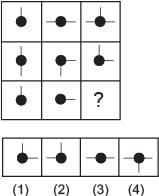Solution:
QUESTION: 4

Select a suitable figure from the four alternatives that would complete the figure matrix.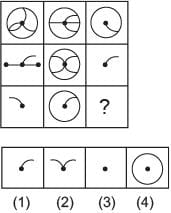Solution:
QUESTION: 5

Select a suitable figure from the four alternatives that would complete the figure matrix.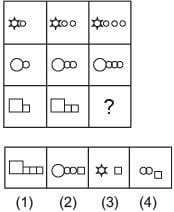Solution:
QUESTION: 6

Select a suitable figure from the four alternatives that would complete the figure matrix.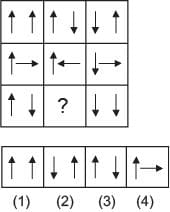Solution:
QUESTION: 7

Select a suitable figure from the four alternatives that would complete the figure matrix.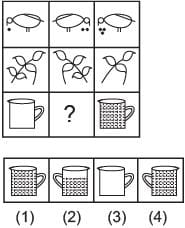Solution:
QUESTION: 8

Select a suitable figure from the four alternatives that would complete the figure matrix.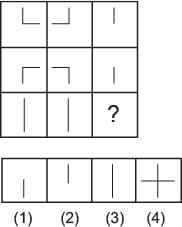Solution:
QUESTION: 9

Select a suitable figure from the four alternatives that would complete the figure matrix.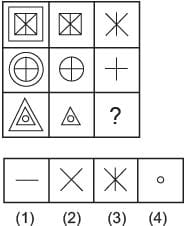Solution:
QUESTION: 10

Select a suitable figure from the four alternatives that would complete the figure matrix.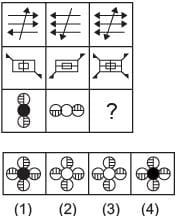Solution:Use Code STAYHOME200 and get INR 200 additional OFF Use Coupon Code